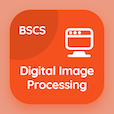Computer Science Courses

Digital Image Processing Exam Prep

Digital Image Processing Practice Test 13

# Wavelet Transforms in One Dimension Quiz Questions PDF - 13

The Book Wavelet Transforms in One Dimension Quiz Questions, wavelet transforms in one dimension MCQ with answers PDF chapter 10-13 to learn online courses, digital image processing tests. Practice Wavelet and Multiresolution Processing trivia questions, wavelet transforms in one dimension Multiple Choice Questions (MCQ Quiz) for online college degrees. The App Wavelet Transforms in One Dimension Quiz e-Book PDF Download: wavelet transforms in one dimension, point line and edge detection, examples of using modalities, power law transformation, line detection in image segmentation test prep for online bachelor's degree computer science.

The Quiz: Wavelet series equation is the sum of PDF, "Wavelet Transforms in One Dimension Quiz" App Download (Free) with detail coefficient, scaling coefficient, span coefficient, and both a and b choices for cheapest online computer science degree. Solve wavelet and multiresolution processing questions and answers, Amazon eBook to download free sample for computer majors.

## Image Processing: Wavelet Transforms in One Dimension Quiz

MCQ: Wavelet series equation is the sum of

A) scaling coefficient
B) detail coefficient
C) span coefficient
D) Both A and B

MCQ: Transition between objects and background shows

A) ramp edges
B) step edges
C) sharp edges
D) Both A and B

MCQ: For marine acquisition the energy source consists usually of

A) five gun towed
B) four gun towed
C) three gun towed
D) two gun towed

MCQ: The correction of power law response is called

A) alpha correction
B) gamma correction
C) beta correction
D) pixel correction

MCQ: Horizontal lines are angles at

A) 0
B) 30
C) 45
D) 90

### Mock Tests: Digital Image Processing Course Prep

The Apps: Digital Image Processing Quiz App, Database Management System MCQs App, and Digital Logic Design MCQ App to download/install for Android & iOS devices. These Apps include complete analytics of real time attempts with interactive assessments. Download Play Store & App Store Apps & Enjoy 100% functionality with subscriptions!